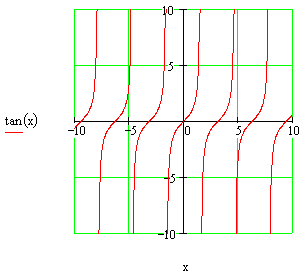# The Graph of the Tangent FunctionTo the left is the graph of the tangent function.

Click the EZ Graph link below to draw this graph with a graphic calculator, explanations included. Links for other examples of the tangent function graph along with their explanations follow below.

More graphs of the tangent function using EZ Graph:

Angle in degrees: